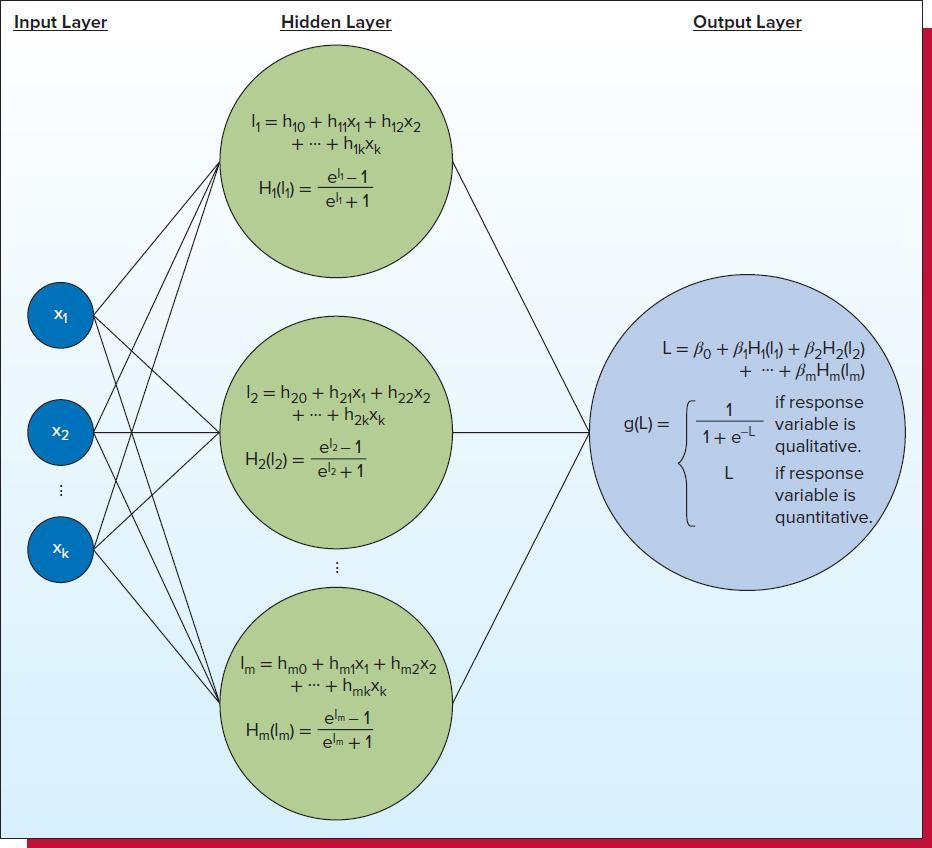Question:

Please answer each of the following questions in detail and provide examples for better clarity, wherever applicable. Provide in-text citations.

Figure 1 shows a typical composition of neurons in a net. This discussion questions aimed at learning how natural composition of neural nets can be mimicked to derive algorithms that can provide prediction at higher levels of complexity.

1. Consider a multiple regression model of your choice containing two predictors. Calculate the values of the regression equation with a range chosen by you for the values of the predictors. Provide the graph of the regression equation which is a plane.

2. Augment your regression model by a sigmoid posterior filter. Calculate the values of the regression equation within the range chosen in part 1. Provide the graph of the regression equation, which will be a sigmoidal surface. Show that this graph cannot have a local extremum.

3. Now, add another regression model with sigmoid posterior containing the same two predictors in the previous part. Repeat parts 1 and 2 for this new regression model.

4. Now consider a model of a neural net, which has two parallel hidden layers, which are the two regression models considered above. The output of the net is simply the superposition of the two regression models. Show that by proper choice of the parameters you can have an output, which has a local extremum within the choice for the range of the values of the predictor. Provide the graph of the regression equation.

5. How does this observation indicate an application of neural networks modeling in practice?

Figure 1

Typical Composition of Neurons in a NetNote:

1. In answering the above questions, you can provide examples formulated according to the model’s setting without actually constructing the solution.

2. Need to have at least 1 peer-reviewed article as the reference and textbook as the reference

3. Need in-text citation

4. Please find the attachments as the power points of the course for reference.

5. Textbook Information:

Bowerman, B., Drougas, A. M., Duckworth, A. G., Hummel, R. M. Moniger, K. B., & Schur, P. J.  (2019). Business statistics and analytics in practice (9th ed.). McGraw-Hill

ISBN 9781260187496

6. Please find the Course Learning Outcome list of this course in the attachment

7. Need to explain in detail and provide examples